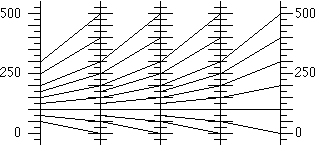# Iterating FunctionsHenri Picciotto

This is a rich topic, which I use to introduce sequences. It is also a good opportunity to introduce subscript notation and limits. It is a necessary beginning to any discussion of dynamical systems.

This work can start at almost any level in high school. It can be distributed into Algebra 1, Algebra 2, and Precalculus, or taught as one unit.

## Linear

Two packets:

1. "Iterating Linear Functions: An Introduction to Dynamical Systems". These are activities which I co-authored with Jonathan Choate for The Mathematics Teacher, February 1997, special issue on Algebra. Use them to introduce these ideas.
Teacher's Guide (PDF)
Student Sheets (PDF)
2. Some lessons from Algebra: Themes, Tools, Concepts, (1994) the book I co-authored with Anita Wah. You can use "Instant Riches" before the above packet, and the rest as a source of additional problems, homework, and assessments. Two PDF files:
Teacher's Guide (PDF)
Student Sheets (PDF)

There is a difference in terminology between the two packets: what is known as a "time series graph" in the first is called "linked function diagrams" in the second.

Both packets require technological support, such as a spreadsheet program, a graphing calculator, Fathom, Desmos, or GeoGebra.

GeoGebra applets:
with a table | with linked function diagrams | with a 'cobweb' graph
Fathom files:
Arithmetic
Linear

Quick review/overview of key concepts, plus derivation of an explicit formula for the nth iterate. (For 11th/12th graders, or teachers.)

I created this applet (Tri, Tri Again) to illustrate a wonderful (and unexpected?) application of these ideas to geometry, by Rachel Chou:
Triangles and Iteration (PDF)

## Non-Linear

After this, we are ready for a more formal approach to the above material, and an exploration of the iteration of some non-linear functions:
See Infinity, Unit 2: Chaos
Finally, a number-theoretic extension:
Iterating f(x)=2|x|-2